# 0.PTA得分截图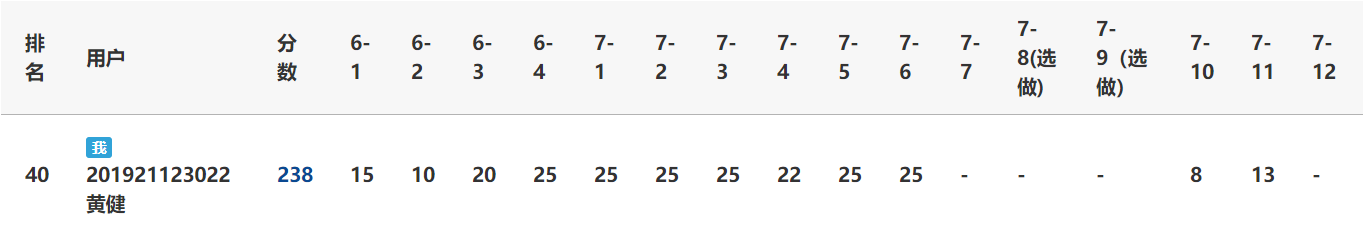# 1.本周学习总结

## 1.1 总结树及串内容

• 串的BF\KMP算法

• 串的BF算法
• BF算法，即暴力(Brute Force)算法，是普通的模式匹配算法，将目标串S的第一个字符与模式串T的第一个字符进行匹配，若相等，则继续比较S的第二个字符和T的第二个字符；若不相等，则比较S的第二个字符和T的第一个字符，依次比较下去，直到得出最后的匹配结果
• 代码
int BF(char* s, char* t)
{
int i = 0, j = 0, countOne = 0, countTwo = 0;
while (s[i] != '\0')
{
i++;
countOne++;  //变量countOne记录目标串s的长度
}
while (t[j] != '\0')
{
j++;
countTwo++;   //变量countTwo记录模式串t的长度
}
i = 0, j = 0;
while (i < countOne && j < countTwo)
{
if (s[i] == t[j])   //若相等，继续比较两串的下一字符
{
i++;
j++;
}
else   //若不相等，目标串s回溯到下一字符处，模式串t回溯到第一个字符处
{
i = i - j + 1;
j = 0;
}
}
if (j >= countTwo)
{
return i - countTwo;
}
else
{
return -1;
}
}

• 串的KMP算法
• KMP算法消除了主串指针的回溯，从而使算法效率提高，其中数组next[]中元素的计算是KMP算法中最核心的部分
• 代码
 int KMP(char* s, char* t)
{
int next;
int i = 0, j = 0;
Getnext(next, t);
int a = 0, countOne = 0, countTwo = 0, b = 0;
while (s[a] != '\0')
{
a++;
countOne++;   //变量countOne记录目标串s的长度
}
while (t[b] != '\0')
{
b++;
countTwo++;   //变量countTwo记录模式串t的长度
}
while (i < countOne && j < countTwo)
{
if (j == -1 || s[i] == t[j])
{
i++;
j++;
}
else
{
j = next[j];    //j回退
}
}
if (j >= countTwo)   //匹配成功，返回子串的位置
{
return (i - countTwo);
}
else
{
return (-1);
}
}
void Getnext(int next[], char* t)
{
int j = 0, k = -1;
next = -1;
int b = 0, countTwo = 0;
while (t[b] != '\0')
{
b++;
countTwo++;
}
while (j < countTwo - 1)
{
if (k == -1 || t[j] == t[k])
{
j++; k++;
if (t[j] == t[k])   //当两个字符相同时，就跳过
{
next[j] = next[k];
}
else
{
next[j] = k;
}
}
else
{
k = next[k];
}
}
}

• 二叉树存储结构、建法、遍历及应用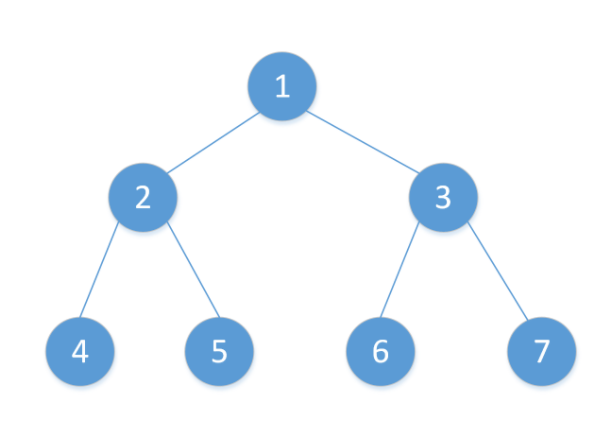• 二叉树存储结构
• 链式存储结构：用链表来表示一棵二叉树，左右指针分别用来表示该节点左孩子和右孩子所在的链节点的存储地址
typedef struct TNode {   //二叉树结点由数据域，左右指针组成
char data;
struct TNode* lchild;
struct TNode* rchild;
}TNode, * BTree;

• 顺序存储结构:用一组连续的存储单元存放二叉树中的节点，用编号的方法从树根起，自上层至下层，每层自左至右地给所有节点编号，但可能对存储空间造成极大的浪费
#define Maxsize 100
typedef struct TNode {
char tree[Maxsize];   //数组存放二叉树中的节点
int parent;  //表示双亲结点的下标
}TNode, * BTree;

• 二叉树建法
• 建树函数的参数为字符串
int n = 0;   //全局变量n，用于每次调用建树函数时访问字符串中的字符
BTree Create(string str)
{
BTree tree;
if (str[n] == '#')   //字符'#'代表空节点
{
n++;
return NULL;
}
else
{
tree = new TNode;   //树结构按照树的先序遍历递归建树
tree->data = str[n];
n++;
tree->lchild = Create(str);
tree->rchild = Create(str);
}
return tree;
}

• 建树函数的参数为字符串和整型变量
BTree Create(string str,int i)
{
BTree tree = new TNode;   //创建根节点
tree->data = str[i];
if (2 * i > str.length() - 1 || str[2 * i] == '#')   //若字符串下标出界或字符为'#'，子树为空
{
tree->lchild = NULL;
}
else
{
tree->lchild = Create(str, 2 * i);   //递归调用建树函数构建子树
}
if (2 * i + 1 > str.length() - 1 || str[2 * i + 1] == '#')
{
tree->rchild = NULL;
}
else
{
tree->rchild = Create(str, 2 * i + 1);
}
return tree;
}

• 二叉树遍历
• 先序遍历（前序遍历）：对于当前节点，先输出该节点，然后输出它的左孩子，最后输出它的右孩子
void PreorderTraverse(BinTree BT)
{
if (BT == NULL)
{
return;
}
else
{
cout << BT->data << " ";   //访问根节点
PreorderTraverse(BT->Left);
PreorderTraverse(BT->Right);
}
}

• 中序遍历：对于当前节点，先输出它的左孩子，然后输出该节点，最后输出它的右孩子
void InorderTraverse(BinTree BT)
{
if (BT == NULL)
{
return;
}
else
{
InorderTraverse(BT->Left);
cout << BT->data << " ";   //访问根节点
InorderTraverse(BT->Right);
}
}

• 后序遍历：对于当前节点，先输出它的左孩子，然后输出它的右孩子，最后输出该节点
void PostorderTraverse(BinTree BT)
{
if (BT == NULL)
{
return;
}
else
{
PostorderTraverse(BT->Left);
PostorderTraverse(BT->Right);
cout << BT->data << " ";   //访问根节点
}
}

• 层次遍历：借助队列，逐层地对二叉树从左到右访问所有节点
void level(BTree tree)
{
queue<BTree>qu;
qu.push(tree);   //根结点入队列
while (!qu.empty())
{
if (qu.front()->lchild != NULL)   //左子树的根结点入队
{
qu.push(qu.front()->lchild);
}
if (qu.front()->rchild != NULL)   //右子树的根结点入队
{
qu.push(qu.front()->rchild);
}
cout << qu.front()->data << " ";
qu.pop();
}
return;
}

• 二叉树应用:基于哈夫曼树（最优二叉树）的哈夫曼编码，可有效缩短编码长度，在数据压缩上有重要应用
• 树的结构及应用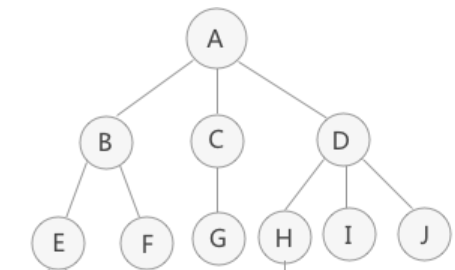• 树的结构：树结构是一种非线性存储结构，存储的是具有“一对多”关系的数据元素的集合

• 树的节点：使用树结构存储的每一个数据元素都被称为“节点”。如上图所示，对A，B，C，D四个节点而言，节点A是节点B，C，D的父节点（双亲节点），节点B，C，D是节点A的孩子节点。且B，C，D三个节点有相同的双亲节点，所以它们互为兄弟节点。每一棵非空树都只有一个根节点，即没有双亲节点的节点。树结构中若一节点没有任何孩子节点，那么此节点称为叶子节点。

• 节点的度：一个节点拥有的子树的个数称为节点的度，如上图中节点A的度为3，而一棵树的度是树内各节点的度的最大值。

• 节点的层次：从一棵树的树根开始，树根所在层为第一层，根的孩子节点所在的层为第二层，依次类推。如上图所示，A 节点在第一层，B，C，D节点在第二层。而一棵树的深度（高度）是树中节点所在的最大的层次。

• 有序树和无序树：如果树中节点的子树从左到右看，谁在左边，谁在右边，是有规定的，这棵树称为有序树；反之称为无序树。在有序树中，一个节点最左边的子树称为"第一个孩子"，最右边的称为"最后一个孩子"。

• 森林：由 n（n >= 1）个互不相交的树组成的集合被称为森林。如上图所示，分别以B，C，D为根节点的三棵子树就可以称为森林。

• 树的存储结构

• 双亲表示法：树中每个节点都有唯一的一个双亲节点，用一组连续的存储空间（一维数组）存储树中的各个节点。树的双亲表示法对于寻找双亲节点和根节点很方便，但是要求某节点的孩子节点，就需要遍历整个数组，而且也不能反映兄弟节点之间的关系，因此要找某节点的兄弟节点也很困难。
#define Maxsize 100
typedef struct TNode{   //节点结构
char data;
int parent;   //节点的双亲在数组中的序号
}TNode;

typedef struct Tree{   // 树结构
PTNode nodes[Maxsize];   // 结点数组
int root;   // 根节点在数组中的序号
int num;   // 树的节点个数
}Tree;

• 孩子表示法：孩子表示法有两种节点结构，即孩子链表的孩子节点和表头数组的表头节点。孩子表示法先把每个节点的孩子节点排列起来，以单链表作为存储结构，再采用顺序存储结构，用一个数组存放n个头指针。
#define Maxsize 100
typedef struct CNode{   //孩子节点结构
int child;   //孩子节点的序号
struct CNode * next;   //指向下一节点的指针
}CNode, *ChildPtr;

char data;   //节点数据
ChildPtr FirstChild;   //指向第一个孩子节点的指针

typedef struct Tree{   //树结构
int root;   //根节点在数组中的序号
int num;   //树的节点个数
}Tree;

• 双亲孩子表示法：将双亲表示法和孩子表示法结合，就形成了双亲孩子表示法
#define Maxsize 100
typedef struct CNode{   //孩子节点结构
int child;   //孩子节点的序号
struct CNode * next;   //指向下一节点的指针
}CNode, *ChildPtr;

char data;   //节点数据
int parent;   //双亲节点的序号
ChildPtr FirstChild;   //指向第一个孩子节点的指针

typedef struct Tree{   //树结构
int root;   //根节点在数组中的序号
int num;   //树的节点个数
}Tree;

• 孩子兄弟表示法：设置两个指针，分别指向节点的第一个孩子和此节点的右兄弟。
typedef struct TNode{
char data;
struct TNode * FirstChild;   //指针指向节点的第一个孩子
struct TNode * Rbrother;   //指针指向节点的右兄弟
}TNode, *Tree;

• 树的应用

• 打开文件夹时，若要出现多个子文件夹，使用的存储结构就是树结构
• QQ联系人的分类中，同一类联系人放到同一目录下，使用的存储结构就是树结构
• 线索二叉树：利用树中原来的空链域存放指针，指向树中其他节点。这种指针称为线索，加上线索的二叉树就称之为线索二叉树

• 线索二叉树节点结构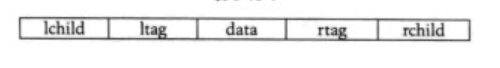typedef struct TNode {
char data;   //节点数据
int ltag，rtag;   //左右标志
struct TNode *lchild;   //左孩子指针
struct TNode *rchild;   //右孩子指针
}TNode,*Tree;


### 如下图所示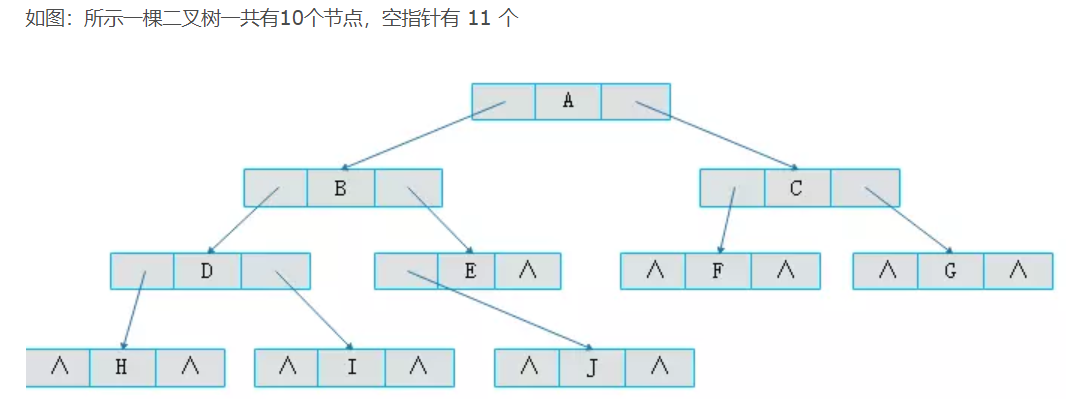• 1.ltag为0时指向该节点的左孩子，为1时指向该节点的前驱
• 2.rtag为0时指向该节点的右孩子，为1时指向该节点的后继

#### 这种指向前驱和后继的指针称为线索。将一棵普通二叉树以某种次序遍历，并添加线索的过程称为线索化，按规则将上图线索化后如下图。此时中序遍历普通二叉树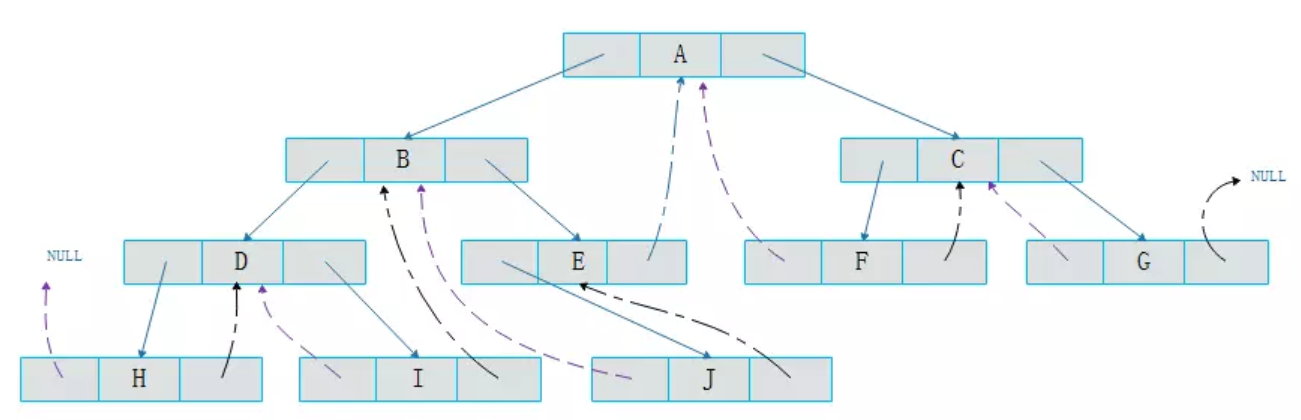#### 图中黑色点画线为指向后继的线索，紫色虚线为指向前驱的线索，其中节点E指向节点A的点画线应为黑色。可以看出通过线索化，既解决了空间浪费的问题，又解决了前驱后继的记录问题。

• 哈夫曼树、并查集
• 哈夫曼树：具有最小带权路径长度的二叉树，也称最优树

• 哈夫曼树节点结构：顺序结构，用数组存放哈夫曼树中的节点
typedef struct HNode {
char data;   //节点值
float weight;   //权重
int parent;   //双亲节点在数组中的序号
int lchild;   //左孩子节点在数组中的序号
int rchild;   //右孩子节点在数组中的序号
}HNode;

• 构造哈夫曼树的过程
1. 对给定的n个权值{W1，W2，...，Wn}，构造n棵二叉树的初始集合F={T1，T2，...，Tn}，其中每棵二叉树Ti中只有一个权值为Wi的根节点，它的左右子树均为空
2. 在集合F中选取根节点的权值最小和次小的两棵二叉树作为左，右子树构造一棵新的二叉树，新二叉树的根节点的权值为其左右子树的根节点的权值之和
3. 从集合F中删除作为左，右子树的两棵二叉树，并把这棵新的二叉树加入到集合F中
4. 重复步骤2和3，直到集合F中只有一棵二叉树为止，这棵二叉树便是要建立的哈夫曼树
• 哈夫曼编码：根节点到叶子节点经过路径组成的0，1序列。左分支用“0”表示，右分支用“1”表示。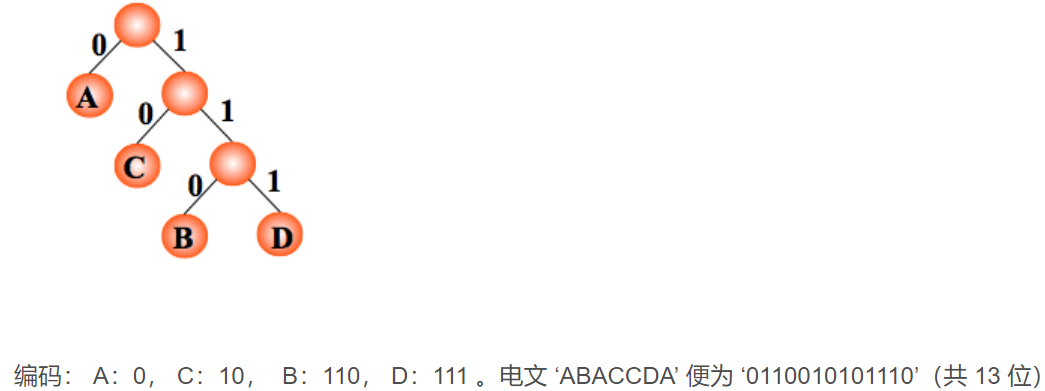• 并查集：一种支持查找一个元素所属的集合及合并2个元素各自专属的集合等运算的数据结构，其存储结构为顺序存储结构

• 节点的结构体
typedef struct UFNode {
int data;   //节点对应元素的编号
int rank;   //节点秩：子树的高度，合并用
int parent;   //双亲节点的下标
}UFNode;


## 1.2.谈谈你对树的认识及学习体会

• 感觉树的学习难了很多，之前学的结构都是线性结构，到了树这一部分变成了非线性结构，结构体中指针个数增加了，节点间的关系也更复杂。但在树的学习中加深了对递归函数的理解，有些实在难搞懂的知识点就自己画简单的树形图来帮助理解。

# 2.阅读代码

## 2.1 找出克隆二叉树中的相同节点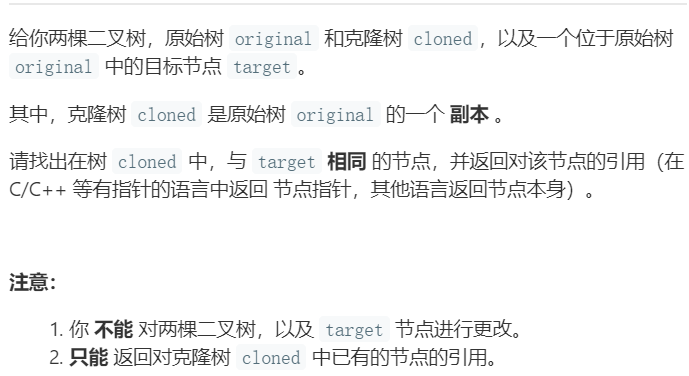• 递归算法
TreeNode* getTargetCopy(TreeNode* original, TreeNode* cloned, TreeNode* target)
{
if (original == NULL) return NULL;
if (target == original) return cloned;
TreeNode* left = getTargetCopy(original->left, cloned->left, target);
TreeNode* right = getTargetCopy(original->right, cloned->right, target);
return left == NULL ? right : left;
}


### 2.1.1 该题的设计思路

• 原始树original和克隆树cloned，两棵树同时访问左孩子节点或右孩子节点，通过原始树original中节点与目标节点target的比较，返回克隆树中相对应的节点
• 算法的时间复杂度T(n)=O(n)，空间复杂度S(n)=O(n)，其中n为二叉树中的节点个数

### 2.1.2 该题的伪代码

TreeNode* getTargetCopy(TreeNode* original, TreeNode* cloned, TreeNode* target)
{
若原始树original为空，return NULL
若原始树original的根节点符合条件，返回克隆树cloned的根节点
同时递归调用函数getTargetCopy()，同时访问两棵树的左孩子节点和右孩子节点
}


### 2.1.3 运行结果### 2.1.4分析该题目解题优势及难点

• 该算法中原始树用来判断访问节点的路径，同时遍历原始树和克隆树，最后巧妙地通过三目运算符来控制返回值，但要注意递归出口的表达

## 2.2 层数最深叶子节点的和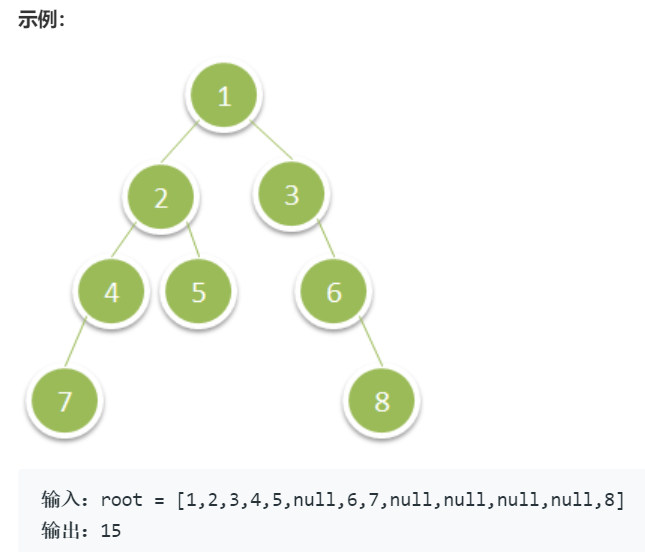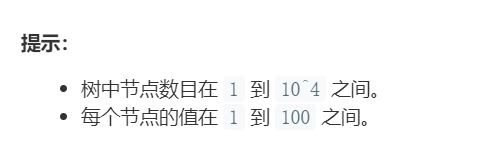• 深度优先搜索
void dfs(TreeNode* node, int dep)
{
if (!node)
{
return;
}
if (dep > maxdep)
{
maxdep = dep;
total = node->val;
}
else if (dep == maxdep)
{
total += node->val;
}
dfs(node->left, dep + 1);
dfs(node->right, dep + 1);
}
int deepestLeavesSum(TreeNode* root)
{
dfs(root, 0);
}


### 2.2.1 该题的设计思路

• 遍历二叉树，通过节点的层次大小比较来进行节点权值的相加，通过递归实现左右子树的遍历
• 该算法的时间复杂度T(n)=O(n)，空间复杂度S(n)=O(1)，其中n为二叉树中的节点个数

### 2.2.2 该题的伪代码

设置两个全局变量maxdep和total，其中maxdep表示搜索到的节点的最大深度，total表示搜索到的深度等于maxdep的节点的权值之和
int deepestLeavesSum(TreeNode* root)
{
调用dfs()函数，实现对二叉树的遍历
返回total值，即为层数最深叶子节点的和
}
void dfs(TreeNode* node, int dep)
{
若二叉树为空，直接return返回
节点node的深度dep小于maxdep，忽略节点node，继续进行搜索

节点node的深度dep等于maxdep，那么我们将节点node的权值添加到total中

节点node的深度dep大于maxdep，将maxdep置为dep，并将total置为节点node的权值
}


### 2.2.3 运行结果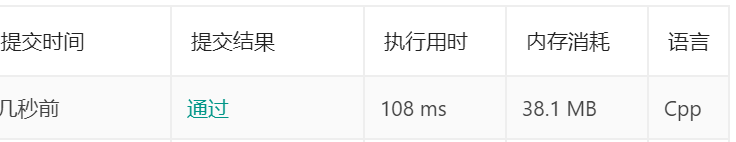### 2.2.4分析该题目解题优势及难点

• 该算法使用了全局变量total，用来表示最终层数最深叶子节点的和。并通过全局变量maxdep，使得每次调用dfs()函数时都能正确地比较节点层次和最大深度的大小，进而进行叶节点权值的相加

## 2.3 二叉搜索树中的插入操作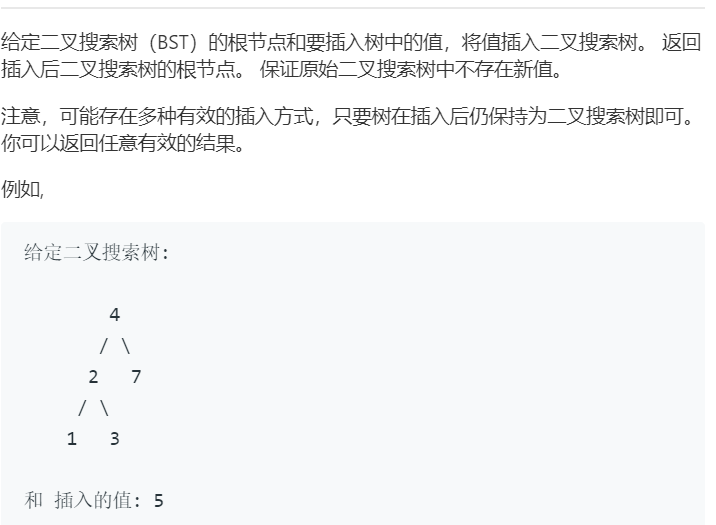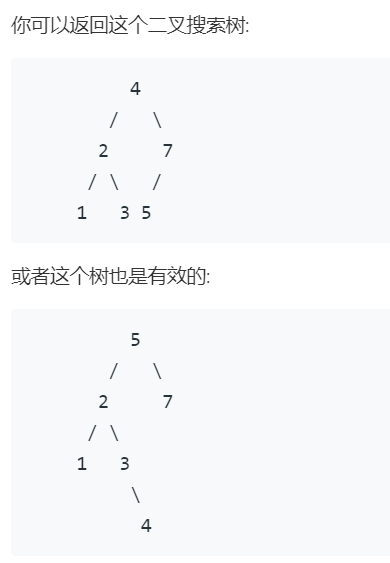• 递归算法
TreeNode* insertIntoBST(TreeNode* root, int val)
{
if (!root)
{
return new TreeNode(val);   //vs调试后，此处应修改根节点的值，并返回根节点
}
if (val < root->val)
{
root->left = insertIntoBST(root->left, val);
}
else
{
root->right = insertIntoBST(root->right, val);
}
return root;
}


### 2.3.1 该题的设计思路

• 先判断树是否为空树，再比较要插入树中的值和根节点值的大小，从而决定要插入的值放在左右子树中的哪一边
• 该算法的时间复杂度T(n)=O(n)，空间复杂度S(n)=O(1)，其中n为树中节点的个数

### 2.3.2 该题的伪代码

TreeNode* insertIntoBST(TreeNode* root, int val)
{
若树为空树，根节点的值为val，返回根节点
若val < root->val，将val作为左子树中的值，插入到左子树中
若val >= root->val，将val作为右子树中的值，插入到右子树中
返回根节点root
}


### 2.3.3 运行结果### 2.3.4分析该题目解题优势及难点

• 该算法利用左孩子节点，根节点，右孩子节点的值依次递增的性质，把val跟根节点的值root->val进行比较，从而确定val值插入到树中的哪一边

## 2.4 两棵二叉搜索树中的所有元素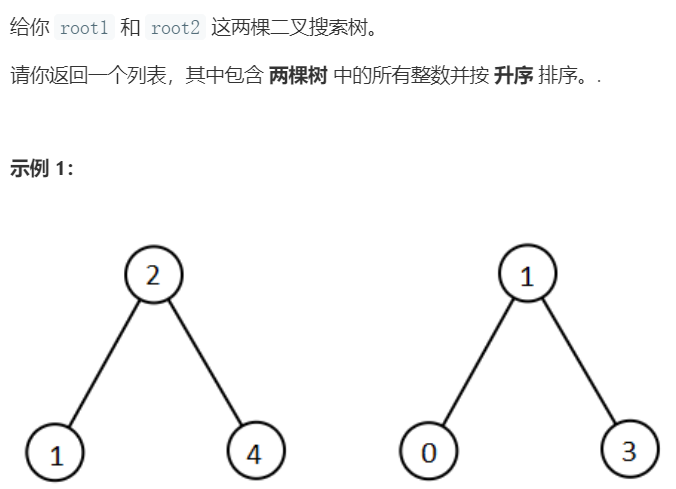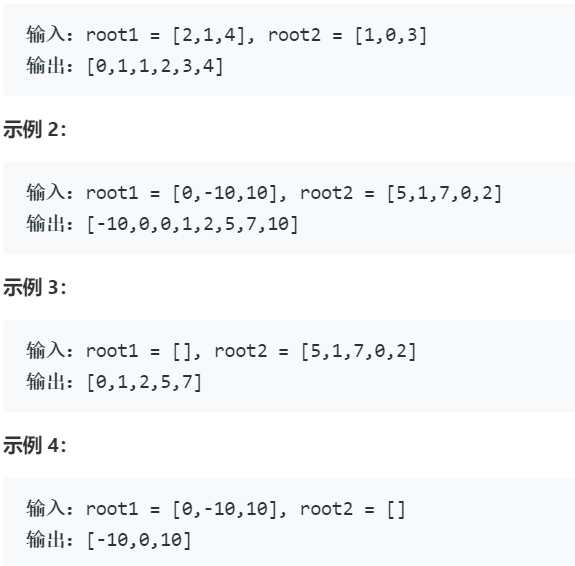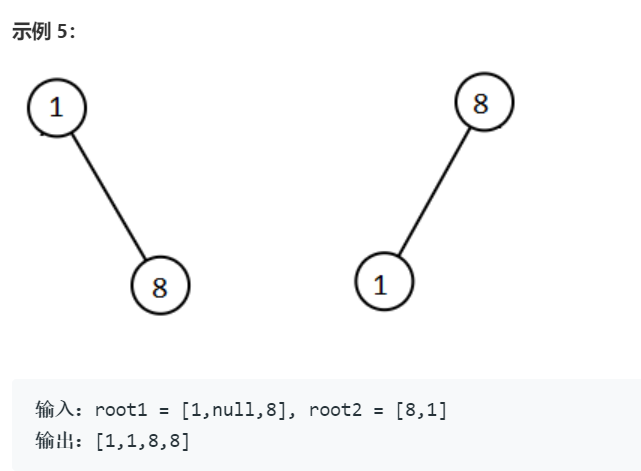• 递归算法，借助vector容器
vector<int> getAllElements(TreeNode* root1, TreeNode* root2)
{
vector<int> ans;
dfs(root1, ans);
dfs(root2, ans);
sort(ans.begin(), ans.end());
return ans;
}

void dfs(TreeNode* node, vector<int>& ans)
{
if (!node)
{
return;
}
ans.push_back(node->val);
dfs(node->left, ans);
dfs(node->right, ans);
}


### 2.4.1 该题的设计思路

• 先把两棵树中所有节点的数据存放到一个数组中，再对这个数组进行排序操作
• 算法的时间复杂度为O((M+N)log(M+N))；空间复杂度为O(H1+H2+log(M+N))，其中 M 和 N 是两棵树中的节点个数，H1和H2是两棵树的高度

### 2.4.2 该题的伪代码

void dfs(TreeNode* node, vector<int>& ans)
{
先序遍历树，把每个节点的数据存入动态数组ans中
}
vector<int> getAllElements(TreeNode* root1, TreeNode* root2)
{
使用vector容器构建动态数组ans
调用dfs()函数，把两棵二叉搜索树中节点的数据存入动态数组ans中
调用vector容器中sort()函数，对动态数组ans中的元素进行从小到大的排序
}


### 2.4.3 运行结果### 2.4.4分析该题目解题优势及难点

• 该算法使用了C++中的vector容器，简化了构建动态数组以及对数组中的元素排序的过程
• 在遍历树时，该算法使用了先序遍历，使用递归的方法来遍历左右子树